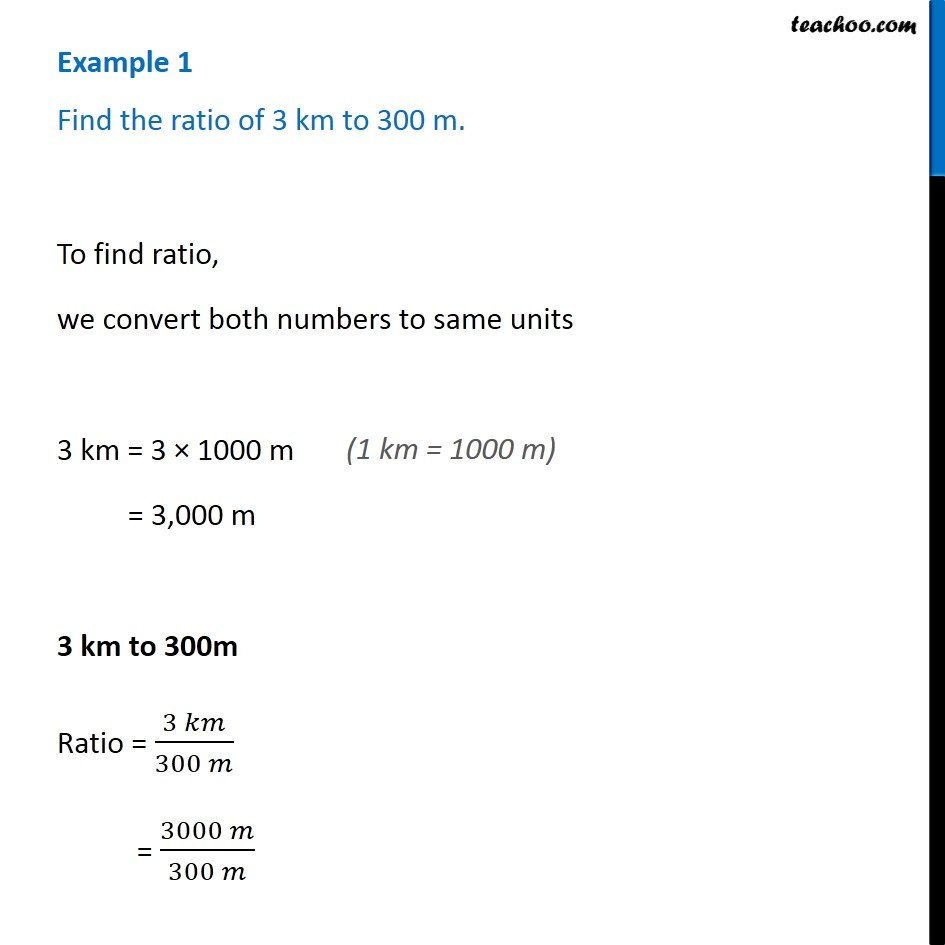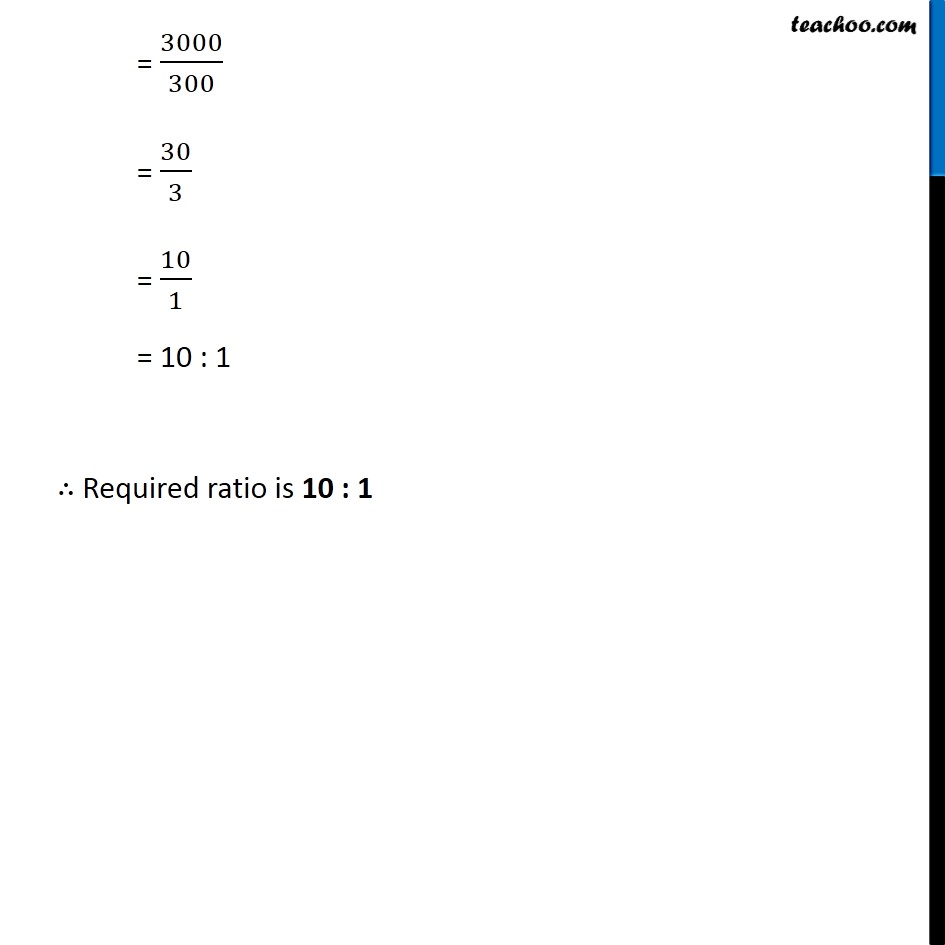Examples

Chapter 7 Class 7 Comparing Quantities
Serial order wiseLearn in your speed, with individual attention - Teachoo Maths 1-on-1 Class

### Transcript

Question 1 Find the ratio of 3 km to 300 m. To find ratio, we convert both numbers to same units 3 km = 3 × 1000 m = 3,000 m 3 km to 300m Ratio = (3 𝑘𝑚)/(300 𝑚) = (3000 𝑚)/(300 𝑚) = 3000/300 = 30/3 = 10/1 = 10 : 1 ∴ Required ratio is 10 : 1